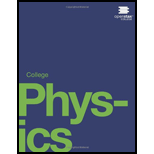# Suppose one load irradiation plant uses a 137 Cs source while another uses an equal activity of 60 Co. Assuming equal fractions of the (rays from the sources are absorbed, why is more time needed to get the same dose using me 137 Cs source?### College Physics

1st Edition
Paul Peter Urone + 1 other
Publisher: OpenStax College
ISBN: 9781938168000### College Physics

1st Edition
Paul Peter Urone + 1 other
Publisher: OpenStax College
ISBN: 9781938168000

#### Solutions

Chapter
Section
Chapter 32, Problem 13CQ
Textbook Problem

## Suppose one load irradiation plant uses a 137Cs source while another uses an equal activity of 60Co. Assuming equal fractions of the (rays from the sources are absorbed, why is more time needed to get the same dose using me 137Cs source?

Expert Solution
To determine

The more time needed to get the same dose using the 137Cs source. Suppose one food irradiation plant uses a 137Cs source while another uses an equal activity of 60Co. Assuming equal fractions of the γ rays from the sources are absorbed.

### Explanation of Solution

Introduction:

To kill the germ present on the food the food radiation is to be used. The radiations passing through the food item so that no waste of the radioactive element on the food items can be achieved.

Such X-rays are used for food radiations which have fewer penetrating rays. Cobalt-60 γ rays has average rate of 1.25 MeV, while 137Cs are of 0.67 MeV . The penetrating rays are created with voltages in the range of 5 million volts and whose photon energies lies up to 5 MeV...

### Want to see the full answer?

Check out a sample textbook solution.See solution

### Want to see this answer and more?

Bartleby provides explanations to thousands of textbook problems written by our experts, many with advanced degrees!

See solution